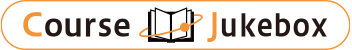### CourseDetailDegree
Bachelor
2 - 4
Course delivery methods
face-to-face
Subject
Social studies, Languages
Program
School
School of Social and International Studies
Department
College of International Studies
Campus
Tsukuba Campus
Classroom
3A305
Course Offering Year
2023-2024
Course Offering Month
April - June
Weekday and Period
Tue1,2
Capacity
Credits
2.0
Language
English
Course Number
BC12061

### Econometrics University of Tsukuba

#### Course Overview

This course is an introduction to econometrics. We will begin with the linear regression model and its estimation and inference. Then we will cover linear models with endogeneity, linear panel models, limited dependent variables, and models used in program evaluations. This course prerequires Introductory Statistics.

#### Learning Achievement

The goal of this course is to understand the fundamental concepts of econometric analysis.

#### Competence

This course is an introduction to econometrics. We will begin with the linear regression model and its estimation and inference. Then we will cover linear models with endogeneity, linear panel models, limited dependent variables, and models used in program evaluations. This course prerequires Introductory Statistics.

1. General-propose competence: critical and creative thinking skills, data and information literacy.
2. Special competence: analytical skills on international relations, analytical skills on international development.

#### Course prerequisites

Introductory Statistics

There will be 3 homework assignments(30%), 1 midterm Exam(30%) and 1 final Exam(40%).

#### Course schedule

This course includes 10 lectures.
Causal inference: potential outcomes, treatment, counterfactuals, selection bias, randomized control experiments, natural (quasi) experiments
Simple linear regressions: identification of parameters, sample analogy estimators, statistical inference
An introduction to the statistic software Stata, (in a computer lab)
Multiple linear regressions: omitted variable bias, control variables, joint tests
Introducing non-linearity into a linear regression: polynomials, logarithmic, dummy independent variables, interactions
Instrumental variable regressions: endogeneity, instrumental variables, examples of valid instrumental variables
Instrumental variable regressions: two stage least square, weak instruments, over-identification
Linear panel models: fixe effects, within estimator, first difference, dynamic panel models
Limited dependent variables: logit and probit
An introduction to program evaluation
Not applicable

Lectures

YU ZHENGFEI

Not applicable

#### Site for Inquiry

Link to the syllabus provided by the university